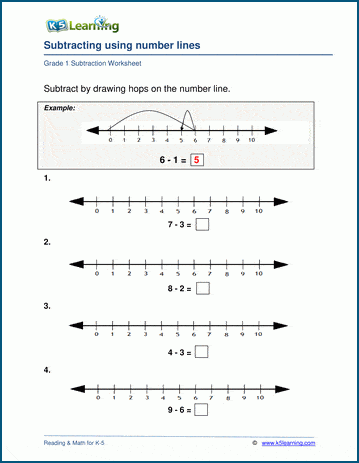# Number Line Subtraction

## Number line subtraction worksheets (numbers < 10, 20)

These grade 1 subtraction worksheets  provide exercises in performing simple subtraction using a number line, and help reinforce the concept of subtraction.  Students solve the problem by drawing subtraction "hops" on the number line to arrive at an answer.## More subtraction worksheets

Explore all of our subtraction worksheets, from subtracting by counting objects to subtracting large numbers in columns.

## What is K5?

K5 Learning offers reading and math worksheets, workbooks and an online reading and math program for kids in kindergarten to grade 5.  We help your children build good study habits and excel in school.